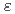The analysis on a density stratification layer consisting of multiple gases in the reactor containment vessel is important for the safety assessment of sever accidents. The Japan Atomic Energy Agency (JAEA) has started the project on the containment thermal hydraulics. We carried out Computational Fluid Dynamics (CFD) analyses in order to investigate the erosion of the density stratification layer by a vertical buoyant jet under this project. We used the Reynolds averaged numerical simulation (RANS) and Large eddy simulation (LES) models to analyze the erosion of a density stratification layer by a vertical buoyant jet in a small vessel which represents a containment vessel. This numerical study calculates the turbulent mixing of a two-component (air and helium) gas mixture. The turbulence models used for the RANS analyses are two types of k-model models. The first model is the low Reynolds number k-model developed by Launder and Sharma. The second model is revised from the first model in order to accurately consider the turbulent production and damping in a stratification layer. The results have indicated that both the RANS and LES models simulate almost the same behavior of the erosion of the density stratification layer. While the erosion rate calculated by the low-Re k-model was faster than that of the LES model, the modified k-model could calculate the erosion rate similar to the LES result.